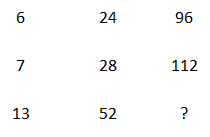Question 5

# Find the missing number from the below options.Solution

Row 1: 6*4 = 24, 24*4 = 96

Row 2: 7*4 = 28, 28*4 = 112

Row 3: 13*4 = 52, 52*4 = 208

Hence option (a) is correct.

• Free SSC Study Material - 18000 Questions
• 230+ SSC previous papers with solutions PDF